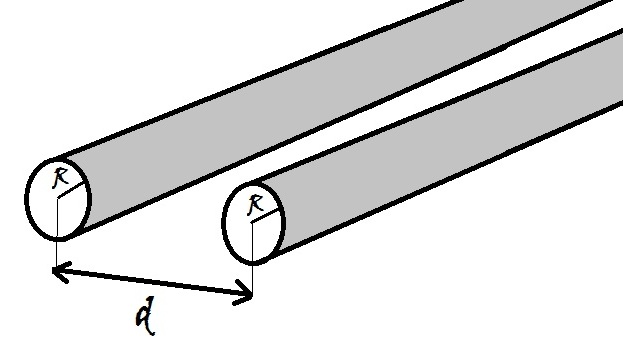# Wire CapacitorDetermine the capacitance per unit length (in $\si[per-mode=symbol]{\farad\per\meter}$) of a system consisting of two long parallel, cylindrical conducting wires of radius $R$ each. The separation between the axes of the wires is $d$.

Details and Assumptions:

• $\displaystyle d = \SI{1}{\milli\meter}$
• $\displaystyle R = \SI{0.4}{\milli\meter}$
• Note that approximating the cylindrical wires as line charges won't work.
×